Clutch Prep is now a part of Pearson
Ch. 1 - A Review of General ChemistryWorksheetSee all chapters

# Degrees of Unsaturation

See all sections
Sections
Intro to Organic Chemistry
Atomic Structure
Wave Function
Molecular Orbitals
Sigma and Pi Bonds
Octet Rule
Bonding Preferences
Formal Charges
Skeletal Structure
Lewis Structure
Condensed Structural Formula
Degrees of Unsaturation
Constitutional Isomers
Resonance Structures
Hybridization
Molecular Geometry
Electronegativity
Isomer
Polar Vs. Nonpolar
Covalent Bond
Polar BondJohnny Betancourt

Degree of unsaturation (Index of Hydrogen Deficiency or IHD) is a measure to how many hydrogen atoms a molecule is missing in order to be fully saturated. A compound that does not have the maximum number of hydrogens per its structure is said to be unsaturated.

## What does it mean for a compound to be saturated?

A molecule that has the maximum number of hydrogen atoms is said to be saturated. A saturated alkane’s molecular formula is as follows: CnH2n+2. For example, hexane (C6H14) is saturated but cyclohexane (C6H12) is not. Hexane and cyclohexane have the exact same number of carbons, but cyclohexane actually has two fewer hydrogens than does hexane.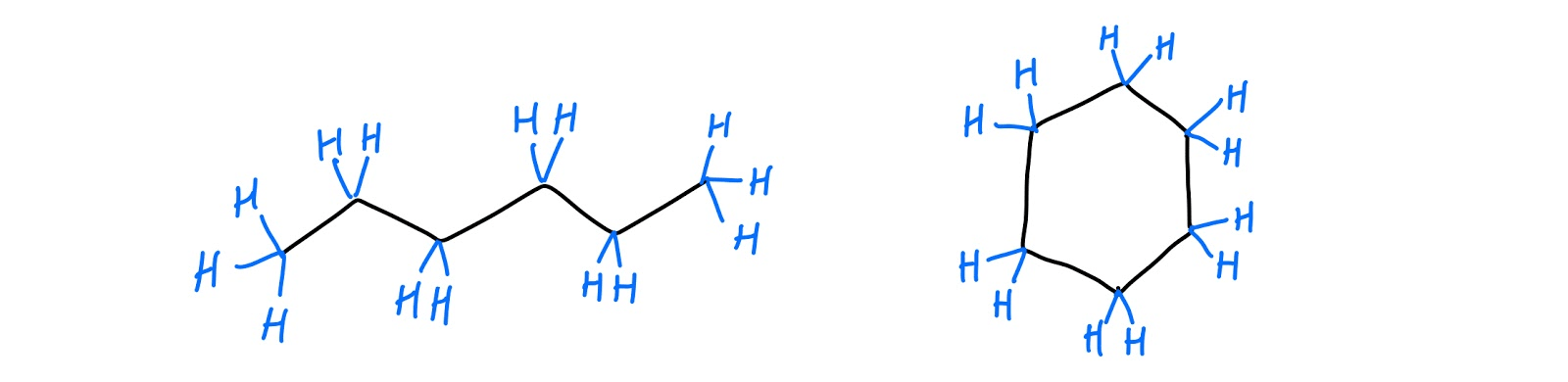hexane (left) and cyclohexane (right)

## How do we determine degrees of unsaturation?

Luckily, determining degrees of unsaturation (AKA index of hydrogen deficiency, hydrogen deficiency index, etc.) isn’t bad at all! There are actually two different ways to do it: 1) when given a molecular formula and 2) when given a structure that’s already drawn-out. Let’s go ahead and break those down below:

### 1. Determining degrees of unsaturation from a molecular formula:

On an exam, you might be given a prompt like this one: “Please provide the degree(s) of unsaturation for each of the following compounds.” Some of those might be molecular formulas like C6H14 or C6H12. For this type of problem, it’s easiest to start with a nice equation. In it, “IHD” will stand for index of hydrogen deficiency (also sometimes represented as DoU).Degrees of Unsaturation general formula

So, now that we’ve got the formula, let’s apply it to our friends hexane and cyclohexane before testing other molecules.IHD hexane formulaIHD cyclohexane formula

### 2. Determining degrees of unsaturation from a structure:

Are you ready for some good news? It turns out that determining IHD from a structure is actually even easier than doing so for a molecular formula. Here’s what you need to know: rings and pi bonds equal 1 IHD (two hydrogens missing). Let’s try working that out with hexane and cyclohexane and see if our results match with those of the formula.IHD of hexane and cyclohexane

Okay, cool! So, even though hexane and cyclohexane have the same number of carbons, cyclohexane has one degree of unsaturation while hexane is completely saturated. Cyclohexane and hexene would have the same IHD since a ring is a double bond equivalent. What would the IHD of cyclohexene be? Cyclohexene would have two degrees of unsaturation because it has a pi bond and a ring.

## Practice:

#### Molecular formula questions:

Calculate the degree of unsaturation in the following formulas.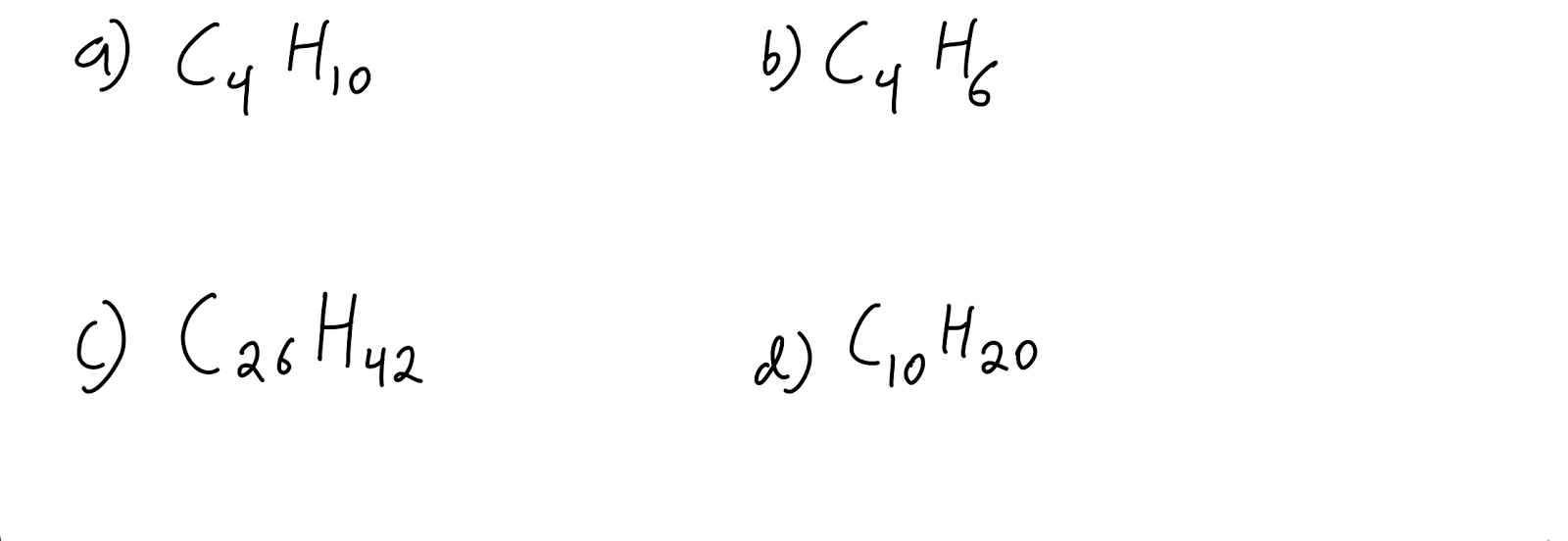Molecular formula blank

#### Structure questions:

How many degrees of unsaturation are present in the molecules drawn below? Let’s look at benzene, naphthalene, 3-hexyne, and styrene.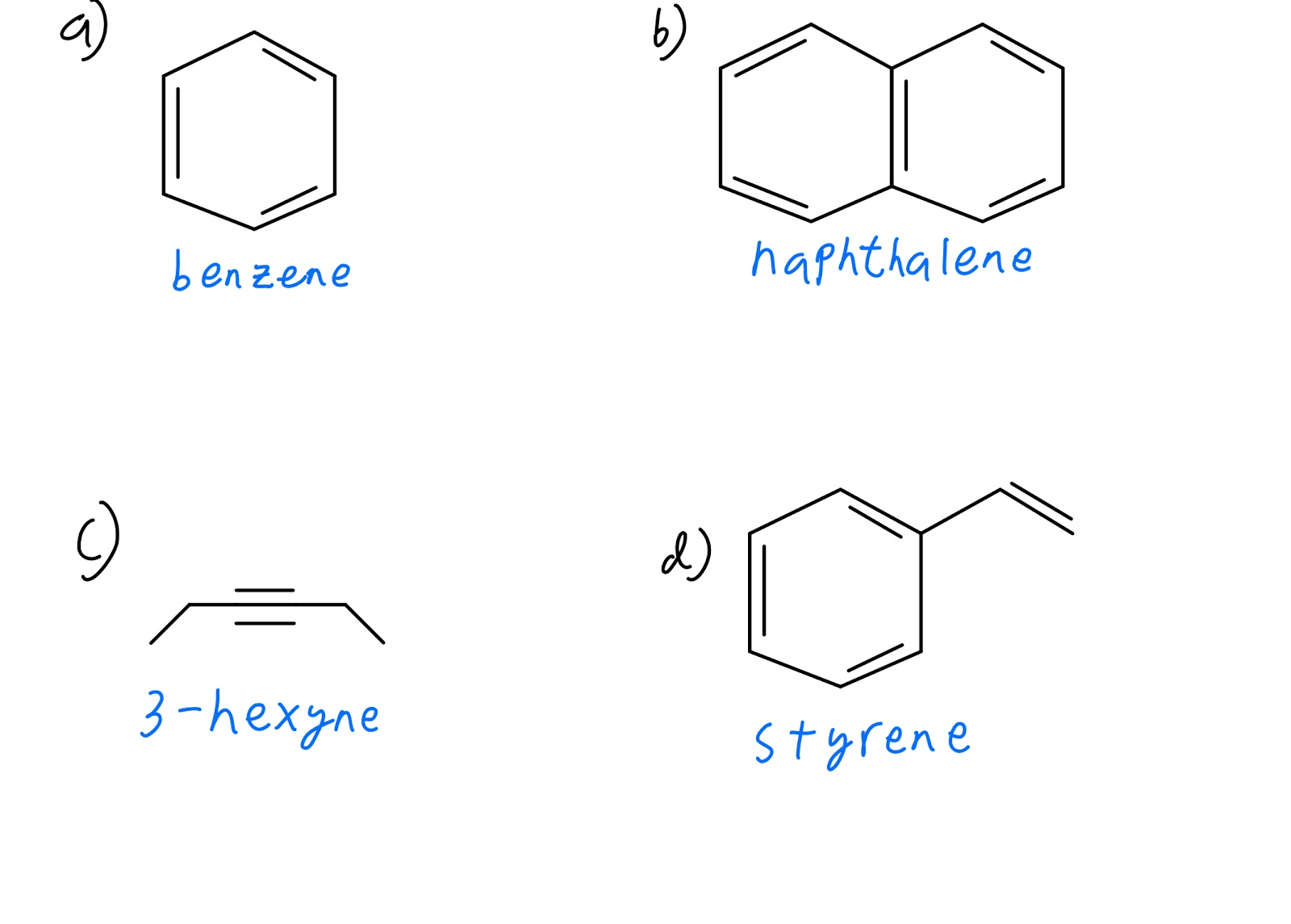Structure question blank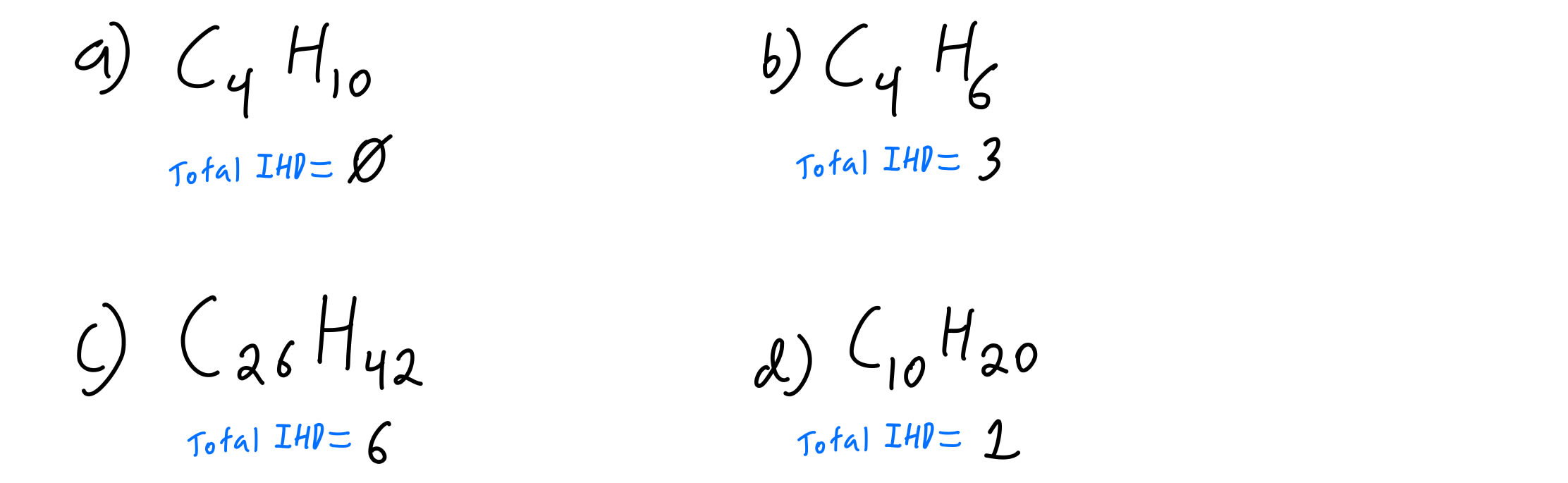Molecular formula answered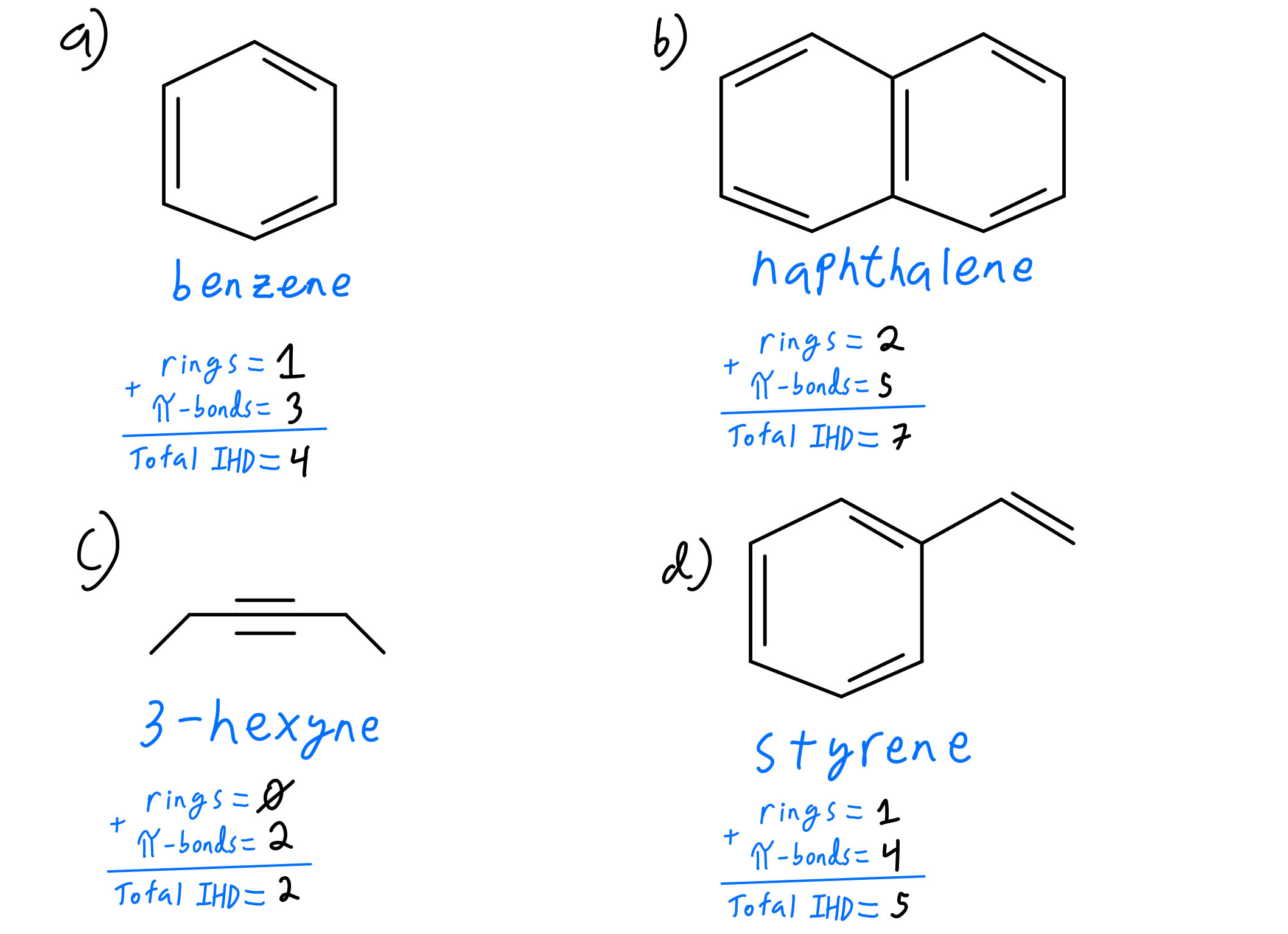Structure questions answeredJohnny Betancourt